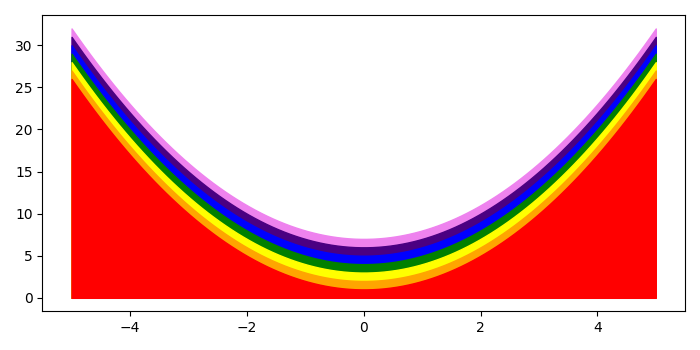# How to fill rainbow color under a curve in Python Matplotlib?

To fill rainbow color under a curve in Python Matplotlib, we can take the following steps −

• Set the figure size and adjust the padding between and around the subplots.
• Create a user-defined method, plot_rainbow_under_curve(), that could have a list of 7 rainbow colors and create a set of data points "x" using numpy.
• Iterate in the range of 0 to 7 and plot the curve and fill the area between that curve.
• To display the figure, use show() method.

## Example

import numpy as np
import matplotlib.pyplot as plt

plt.rcParams["figure.figsize"] = [7.00, 3.50]
plt.rcParams["figure.autolayout"] = True
def plot_rainbow_under_curve():
rainbow_colors = ['violet', 'indigo', 'blue', 'green', 'yellow', 'orange', 'red']
x = np.linspace(-5, 5, 100)
for i in range(0, 7):
plt.plot(x, x ** 2, lw=0)
plt.fill_between(x, x ** 2 + len(rainbow_colors)-i,color=rainbow_colors[i])

plot_rainbow_under_curve()

plt.show()

## Output

It will produce the following output